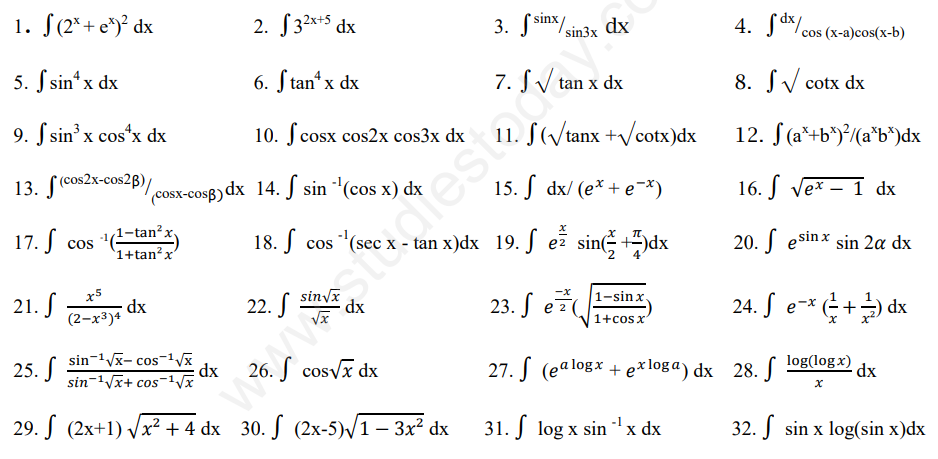# CBSE Class 12 Mathematics Indefinite and Definite Integrals Assignment Set A

Read and download free pdf of CBSE Class 12 Mathematics Indefinite and Definite Integrals Assignment Set A. Get printable school Assignments for Class 12 Mathematics. Standard 12 students should practise questions and answers given here for Chapter 7 Integrals Mathematics in Grade 12 which will help them to strengthen their understanding of all important topics. Students should also download free pdf of Printable Worksheets for Class 12 Mathematics prepared as per the latest books and syllabus issued by NCERT, CBSE, KVS and do problems daily to score better marks in tests and examinations

## Assignment for Class 12 Mathematics Chapter 7 Integrals

Class 12 Mathematics students should refer to the following printable assignment in Pdf for Chapter 7 Integrals in standard 12. This test paper with questions and answers for Grade 12 Mathematics will be very useful for exams and help you to score good marks

### Chapter 7 Integrals Class 12 Mathematics Assignment

CBSE Class 12 Mathematics Indefinite and Definite Integrals Assignment Set A.Chapter wise assignments are being given by teachers to students to make them understand the chapter concepts. Its extremely critical for all CBSE students to practice all assignments which will help them in gaining better marks in examinations. All assignments available for free download on the website are developed by the best teachers having many years of teaching experience in CBSE schools all over the country. Students, teachers and parents are advised to download the CBSE study material.Please click the below link to access CBSE Class 12 Mathematics Indefinite and Definite Integrals Assignment Set A

 CBSE Class 12 Mathematics Indefinite and Definite Integrals Assignment Set A CBSE Class 12 Mathematics Indefinite and Definite Integrals Assignment Set B

## More Study Material

Where can I download in PDF assignments for CBSE Class 12 Mathematics Chapter 07 Integrals

You can download free Pdf assignments for CBSE Class 12 Mathematics Chapter 07 Integrals from StudiesToday.com

The assignments for Chapter 07 Integrals Class 12 Mathematics for have been made based on which syllabus

The Chapter 07 Integrals Class 12 Mathematics Assignments have been designed based on latest CBSE syllabus for Class 12 Mathematics issued for the current academic year

Can I download and print these printable assignments for Mathematics Chapter 07 Integrals Class 12

Yes, These printable assignments for Chapter 07 Integrals Class 12 Mathematics are free to download and print

How many topics are covered in Chapter 07 Integrals Mathematics assignments for Class 12

All topics given in Chapter 07 Integrals Mathematics Class 12 Book for the current academic year have been covered in the given assignment

Is there any charge for this assignment for Chapter 07 Integrals Mathematics Class 12

No, all Printable Assignments for Chapter 07 Integrals Class 12 Mathematics have been given for free and can be downloaded in Pdf format

How can I download the printable test assignments for Chapter 07 Integrals Mathematics Class 12

Just click on the View or Download button below, then another window with the Pdf will be visible, just click on the Pdf icon to download the free assignments for Chapter 07 Integrals Class 12 Mathematics

Are these assignments available for all chapters in Class 12 Mathematics

Yes, apart from Mathematics you can download free assignments for all subjects in Class 12

Can I download solved assignments for Chapter 07 Integrals CBSE Class 12 Mathematics

Our team of expert teachers at studiestoday.com have provided all answers for the practice questions which have been given in Class 12 Mathematics Chapter 07 Integrals assignments